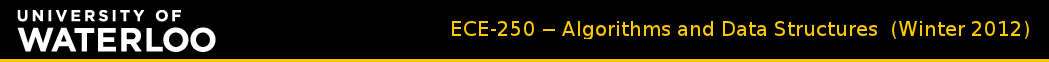Skip to the content of the web site.

# Homework Assignments

 Assignment Suggested Solutions Grades Formula for ID “scrambling” Assignment 1 (due Jan 13, in class) A1 solutions A1 grades Take last 6 digits of student ID; multiply times 117; take last 7 digits of result Assignment 2 (due Jan 27, in class) A2 solutions A2 grades Take last 6 digits of student ID; multiply times 211; take last 7 digits of result Assignment 3 (due Feb 10, in class) A3 solutions A3 grades Take last 6 digits of student ID; multiply times 179 take last 7 digits of result Assignment 4 (due Mar 16, in class) A4 solutions A4 grades Take last 6 digits of student ID; multiply times 191; take last 7 digits of result Assignment 5 (due Mar 30, in class) A5 solutions A5 grades Take last 6 digits of student ID; multiply times 223; take last 7 digits of result